# GSEB Solutions Class 12 Maths Chapter 13 Probability Ex 13.5

Gujarat Board GSEB Textbook Solutions Class 12 Maths Chapter 13 Probability Ex 13.5 Textbook Questions and Answers.

## Gujarat Board Textbook Solutions Class 12 Maths Chapter 13 Probability Ex 13.5

Question 1.
A dice is thrown 6 times. “If getting an odd number” is a success, what is the probability of
(i) 5 successes?
(ii) At least 5 successes?
(iii) At most 5 successes?
Solution:
There are 3 odd numbers on a dice.
∴ Probability of getting an odd numnber on a dice = $$\frac{3}{6}$$ = $$\frac{1}{2}$$.
(i) Probability of getting 5 successes
P(5) = 6C5($$\frac{1}{2}$$)($$\frac{1}{2}$$)5
= 6.($$\frac{1}{2}$$)6 = $$\frac{6}{24}$$ = $$\frac{3}{32}$$.

(ii) Probability of getting at least 5 successes
= P(at least 5 successes)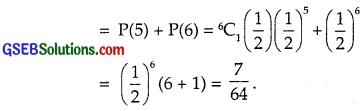(iii) P(at most 5 successes)
= P(0) + P(1) + P(2) + P(3) + P(4) + P(5)
= [P(0) + P(1) + P(2) + P(3) + P(4) + P(5)] – P(6)
= 1 – P(6) = 1 – ($$\frac{1}{2}$$)6 = 1 – $$\frac{1}{64}$$ = $$\frac{63}{64}$$.Question 2.
A pair of dice is thrown 4 times. If getting a doublet is considered a success, find the probability of two successes.
Solution:
When a pair of dice is thrown, a doublet may be obtained in 6 ways.
Total number of exhaustive cases = 36.
∴ Probability of getting a doublet = $$\frac{6}{36}$$ = $$\frac{1}{6}$$.
∴ p = $$\frac{1}{6}$$ and q = $$\frac{5}{6}$$.
In 4 throws, probability of getting two successes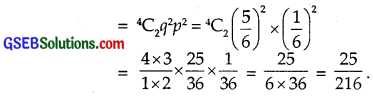Question 3.
There are 5% defective items in a large bulk of items. What is the probability that a sample of 10 items will include not more than one defective item?
Solution:
Probability of getting one defective item
= 5% = $$\frac{5}{100}$$ = $$\frac{1}{20}$$.
Probability of getting a good item = 1 – $$\frac{1}{20}$$ = $$\frac{19}{20}$$.
A sample of 10 items includes not more than one defective item
⇒ Sample contains at most one defective item
Its probability = P(0) + P(1)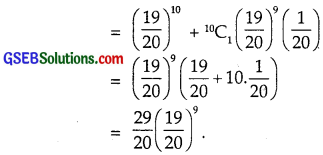Question 4.
Five cards are drawn successively with replacement from a well shuffled deck of 52 cards. What is the probability that
(i) all five cards are spades?
(ii) only three cards are spades?
Solution:
Let one card drawn be a spade.
∴ Its probability = $$\frac{13}{52}$$ = $$\frac{1}{4}$$.
∴ Probability that the cards drawn is not a spade
= 1 – $$\frac{1}{4}$$ = $$\frac{3}{4}$$.

(i) P(all cards drawn are spades)
= ($$\frac{1}{4}$$)5 = $$\frac{1}{1024}$$.

(ii) P(only three cards are spades)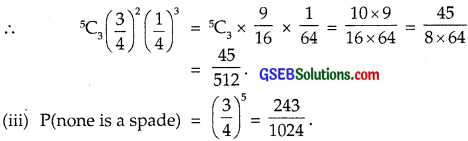Question 5.
The probability that a bulb produced by a factory will fuse after 150 days of use is 0.05. Find the probability that out of 5 such bulbs,
(i) none
(ii) not more than one
(iii) more than one
(iv) at least one will fuse after 150 days of use.
Solution:
Probability that a bulb gets fuse after 150 days of its use.
= 0.05.
∴ Probability that the bulb will not fuse after 150 days of its use
= 1 – 0.05 = 0.95.

(i) Probability that no bulb will fuse after 150 days of its use
= P(none) = (0.95)5
= 0.7738 = 0.77
= 0.77 (approx.).

(ii) P(not more than one)
= P(0) + P(1)
= (0.95)5 + 5C1 × (0.95)4 × (0.05)
= (0.95)4[0.95 + 5 × 0.05]
= (0.95)4(0.95 + 0.25)
= (0.95)4 × 1.2.

(iii) P(more than one) = P(2) + P(3) + P(4) + P(5)
= [P(0) + P(1) + P(2) + P(3) + P(4) + P(5)] – [P(0) + P(1)]
= 1 – [P(0) + P(1)]
= 1 – (0.95)4 × 1.2 [see part (ii)]

(iv) P(at least one) = P(1) + P(2) + P(3) + P(4) + P(5)
= 1 – P(0)
= 1 – (0.95)5 [From (i)]

Question 6.
A bag consists of 10 bulbs each marked with one of the digits 0 to 9. If four bulbs are drawn successively with replacement from the bag, what is the probability that none is marked with digit 0?
Solution:
A bag contains 10 bulbs marked 0 – 9.
Probability of getting a bulb marked with 0
= $$\frac{1}{10}$$ = 0.1.
Probability that the bulb marked 0 is not obtained
= 1 – 0.1 = 0.9
Probability that the bulb marked 0 is not obtained.
Now, 4 bulbs are drawn.
Probability that none is marked with 0
= (0.9)4 = ($$\frac{9}{10}$$)4.Question 7.
In an examination, 20 true-false type questions are asked. Suppose a student tosses a fair coin to determine his answer to each question.
If the coin falls head, he answer ”true’, if it falls tail, he answers ‘false’. Find the probability that he answers at least 12 questions correctly.
Solution:
Probability that student answers a question true = $$\frac{1}{2}$$
i.e., when a coin is thrown, probability that a head is obtained = $$\frac{1}{2}$$.
Probability that his answer is false = 1 – $$\frac{1}{2}$$ = $$\frac{1}{2}$$.
Probability that he answers at least 12 questions correctly
= P(12) + P(13 + P(14) + ………………… + P(20)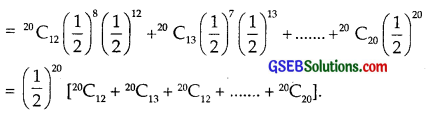Question 8.
Suppose X has a binomial distribution B (6, $$\frac{1}{2}$$), show that X = 3 is mot likely outcome.
Solution: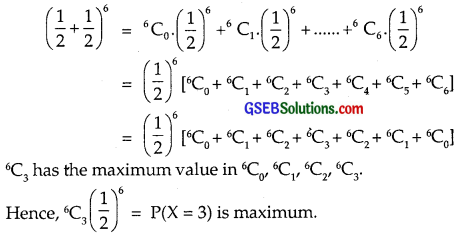⇒ P(X = 3) is most likely outcome.

Question 9.
On a multiple choice examination with three possible answers for each of the five questions, what is the probability that a candidate would get four or more correct answers, just by guessing?
Solution:
One option out of three is correct.
Probability that the answer is correct, i.e., p = $$\frac{1}{3}$$.
Probability that the answer is incorrect = 1 – $$\frac{1}{3}$$ = $$\frac{2}{3}$$ = q.
Probability that four or more answers are correct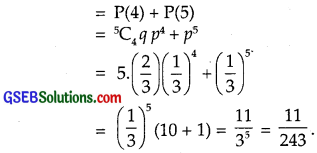Question 10.
A person buys a lottery ticket in 50 lotteries, in each of which his chance of winning a prize is $$\frac{1}{100}$$. What is the probability that he will win a prize
(a) at least once?
(b) exactly once?
(c) at least twice?
Solution:
Probability that the person wins the prize = $$\frac{1}{100}$$
Probability of losing = 1 – $$\frac{1}{100}$$ = $$\frac{99}{100}$$

(a) Probability if he loses in all the lotteries = ($$\frac{99}{100}$$)50
= 1 – ($$\frac{99}{100}$$)50
= 1 – (0.99)50

(b) Probability that he wins exactly once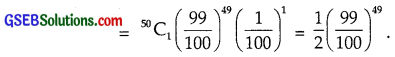(c) Probability that he wins at least twice
= P(2) + P(3) + …. + P(50)
= [P(0) + P(1) +…. + P(50)] – [P(0) + P(1)]
= 1 – [P(0) + P(1)]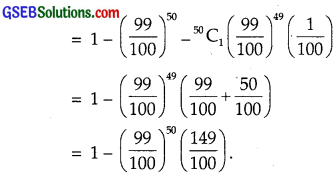Question 11.
Find the probability of getting 5 exactly twice in 7 throws of a dice.
Solution:
When a dice is thrown, the probability of getting a 5 is $$\frac{1}{6}$$.
∴ Probability of not getting 5 is 1 – $$\frac{1}{6}$$ = $$\frac{5}{6}$$.
∴ Probability of getting 5 exactly twice in 7 throws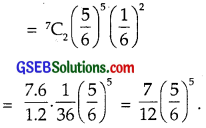Question 12.
Find the probability of throwing at most 2 sixes in 6 throws of a single dice.
Solution:
When a dice is thrown, probability of getting a six = $$\frac{1}{6}$$.
∴ Probability of not getting a six = 1 – $$\frac{1}{6}$$ = $$\frac{5}{6}$$.
Probability of getting at most 2 sixes in 6 throws of a single dice.
= P(0) + P(1) + P(2)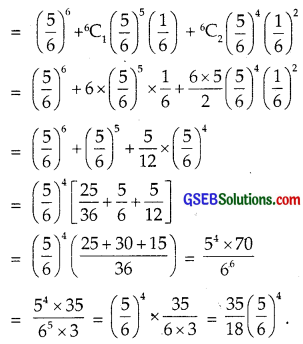Question 13.
It is known that 10% of a certain articles manufactured are defective. What is the probability that in a random sample of 12 such articles, 9 are defective.
Solution:
Probability that the article selected is defective.
= 10% = $$\frac{1}{10}$$.
∴ Probability that the article selected is good = 1 – $$\frac{1}{10}$$ = $$\frac{9}{10}$$.
Probability that in a sample of 12 articles, 9 are defective.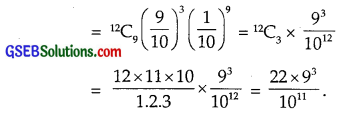In each of the following, choose the correct answer:

Question 14.
In a box containing 100 bulbs, 10 are defective. The probability that out of a sample of 5 bulbs, none is defective is
(A) 10-1
(B) ($$\frac{1}{2}$$)5
(C) ($$\frac{9}{10}$$)5
(D) $$\frac{9}{10}$$)
Solution:
Number of bulbs in the box = 100
Number of defective bulbs = 10
∴ Probability of occuring a defective bulb = $$\frac{10}{100}$$ = $$\frac{1}{10}$$.
⇒ Probability of occurrence of a good bulb = 1 – $$\frac{1}{10}$$ = $$\frac{9}{10}$$.
In a sample of 5 bulbs, the distribution of defective bulbs
= ($$\frac{9}{10}$$ + $$\frac{1}{10}$$)5
Probability that no bulb is defective in this sample = ($$\frac{9}{10}$$)5
⇒ Part (C) is the correct answer.Question 15.
The probability that a student is not a swimmer is $$\frac{1}{5}$$. Then, the probability that out of five students, four are swimmers is
(A) 5C4($$\frac{4}{5}$$)5($$\frac{1}{5}$$)
(B) ($$\frac{4}{5}$$)4$$\frac{1}{5}$$
(C) 5C1$$\frac{1}{5}$$($$\frac{4}{5}$$)4
(D) None of these
Solution:
Probability that a student is not a swimmer = $$\frac{1}{5}$$.
⇒ Probability that a student is a swimmer = 1 – $$\frac{1}{5}$$ = $$\frac{4}{5}$$.
So, probability distribution of students who are swimmers
= ($$\frac{1}{5}$$ + $$\frac{4}{5}$$)5
⇒ Probability that out of 5 students 4 are swimmers
= 5C4($$\frac{1}{5}$$)1($$\frac{4}{5}$$)4
⇒ Part (A) is the correct answer.